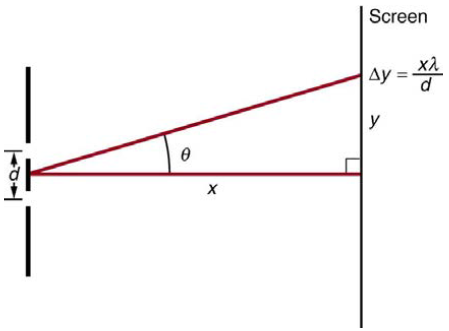Chapter 27, Problem 18PE

Chapter
Section
Textbook Problem

Figure 27.56 shows a double slit located a distance x from a screen, with the distance from the center of the screen given by y. When the distance d between the slits is relatively large, there will be numerous bright spots, called fringes. Show that, for small angles (where sin θ ≈ θ , with θ in radians), the distance between fringes is given by Δ y = x λ / d .Figure 27.56 The distance between adjacent fringes is Δ y = x λ / d , assuming the slit separation d is large compared with λ .

To determine

To Show:

The distance between fringes is given by Δy=xλd

Explanation

Given info:

Figure shows a double slit located a distance x, from a screen, with the distance from a screen, with the distance from the center of the screen given by y . When the distance d between the slits is relatively large, there will be numerous bright spots.

Formula used:

For two adjacent fringes we have,

dsinθm=mλanddsinθm+1=(m+1)λ

Calculation:

Subtracting these equations gives

dsinθm=mλandd(sinθm+1sinθ)=[(m+1)m]

Still sussing out bartleby?

Check out a sample textbook solution.

See a sample solution

The Solution to Your Study Problems

Bartleby provides explanations to thousands of textbook problems written by our experts, many with advanced degrees!

Get Started